# Selina solutions for Concise Physics Class 8 ICSE chapter 8 - Electricity [Latest edition]

#### Chapters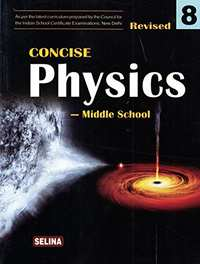## Chapter 8: Electricity

Exercise 8 (A) (Objective questions)OthersExercise 8 (A) (Short/Long answer questions)Exercise 8 (A) (Numericals)Exercise 8 (B) (Objectives question)Exercise 8 (B) (Short/Long answer questions)
Exercise 8 (A) (Objective questions) [Pages 150 - 151]

### Selina solutions for Concise Physics Class 8 ICSE Chapter 8 Electricity Exercise 8 (A) (Objective questions) [Pages 150 - 151]

Exercise 8 (A) (Objective questions) | Q 1.01 | Page 150

A fuse wire has a high melting point.

• True

• False

Exercise 8 (A) (Objective questions) | Q 1.02 | Page 150

Flow of protons constitutes electric current.

• True

• False

Exercise 8 (A) (Objective questions) | Q 1.03 | Page 150

Silver is an insulator of electricity.

• True

• False

Exercise 8 (A) (Objective questions) | Q 1.04 | Page 150

S.I. unit and commercial unit of electrical energy are same.

• True

• False

Exercise 8 (A) (Objective questions) | Q 1.05 | Page 150

• True

• False

Exercise 8 (A) (Objective questions) | Q 1.07 | Page 150

All metals are insulators of electricity.

• True

• False

Exercise 8 (A) (Objective questions) | Q 1.08 | Page 150

The earth wire protects us from an electric shock.

• True

• False

Exercise 8 (A) (Objective questions) | Q 1.09 | Page 150

A switch should not be touched with wet hands.

• True

• False

Exercise 8 (A) (Objective questions) | Q 1.1 | Page 150

All electrical appliances in a household circuit work at the same voltage.

• True

• False

Exercise 8 (A) (Objective questions) | Q 1.11 | Page 150

In a cable, the green wire is the live wire.

• True

• False

Exercise 8 (A) (Objective questions) | Q 1.12 | Page 150

A fuse is connected to the live wire.

• True

• False

Exercise 8 (A) (Objective questions) | Q 1.13 | Page 150

A switch is connected to the neutral wire.

• True

• False

Q 1.6 | Page 150

Our body can pass electricity through it.

• True

• False

Exercise 8 (A) (Objective questions) | Q 2.1 | Page 150

The unit in which we pay the cost of electricity is ..........

Exercise 8 (A) (Objective questions) | Q 2.2 | Page 150

The electrical energy consumed in a house is measured by ...............

Exercise 8 (A) (Objective questions) | Q 2.3 | Page 150

In a household electrical circuit, the appliance are connected in ............with the mains.

Exercise 8 (A) (Objective questions) | Q 2.4 | Page 150

A switch is connected to the .......... wire.

Exercise 8 (A) (Objective questions) | Q 2.5 | Page 150

The red colour insulated wire in a cable is the .............. wire.

Exercise 8 (A) (Objective questions) | Q 2.6 | Page 151

One kilowatt hour is equal to ............ joule.

Exercise 8 (A) (Objective questions) | Q 2.7 | Page 151

A fuse wire should have low .................

Exercise 8 (A) (Objective questions) | Q 3 | Page 151

Match the following

 Column A Column B Electric power volt kWh joule Electric current volt × ampere Electric energy watt watt ampere potential difference electrical energy
Exercise 8 (A) (Objective questions) | Q 4.1 | Page 151

All wires used in electric circuits should be covered with

• colouring material

• conducting material

• an insulating material

• none of the above

Exercise 8 (A) (Objective questions) | Q 4.2 | Page 151

Electric work done per unit time is ____________.

• electrical energy

• electric current

• electric voltage

• electrical power

Exercise 8 (A) (Objective questions) | Q 4.3 | Page 151

One kilowatt is equal to

• 100 watt

• 1000 watt

• 10 watt

• none of these

Exercise 8 (A) (Objective questions) | Q 4.4 | Page 151

Fuse wire is an alloy of

• tin-copper

Exercise 8 (A) (Objective questions) | Q 4.5 | Page 151

A fuse wire should have

• a low melting point

• high melting point

• very high melting point

• none of the above

Exercise 8 (A) (Objective questions) | Q 4.6 | Page 151

When the switch of an electric appliance is put off, it disconnects

• the live wire

• the neutral wire

• the earth wire

• the live and the neutral wire

Exercise 8 (A) (Objective questions) | Q 4.7 | Page 151

The purpose of an electric meter in a house is

• to give the cost of electricity directly

• to give the consumption of electrical energy

• to safeguard the circuit from short circuiting

• to put on or off the mains.

Exercise 8 (A) (Objective questions) | Q 4.8 | Page 151

If out of the two lighted bulbs in a room, one bulb suddenly fuses, then

• other bulb will glow more

• other bulb will glow less

• other bulb will also fuse

• other bulb will remain lighted unaffected.

Exercise 8 (A) (Short/Long answer questions) [Pages 151 - 152]

### Selina solutions for Concise Physics Class 8 ICSE Chapter 8 Electricity Exercise 8 (A) (Short/Long answer questions) [Pages 151 - 152]

Exercise 8 (A) (Short/Long answer questions) | Q 1 | Page 151

From where does the electricity come to our home ?

Exercise 8 (A) (Short/Long answer questions) | Q 2 | Page 151

What is an electric meter? Where is it fixed in our house?

Exercise 8 (A) (Short/Long answer questions) | Q 3 | Page 151

State the purpose of kWh meter.

Exercise 8 (A) (Short/Long answer questions) | Q 4

For which unit do we pay our electricity bill?

Exercise 8 (A) (Short/Long answer questions) | Q 5 | Page 151

How can you check just by seeing the meter whether the electricity is in use or not?

Exercise 8 (A) (Short/Long answer questions) | Q 6 | Page 151

The diagram below in figure shows the reading on the dials of a meter. State what is its reading.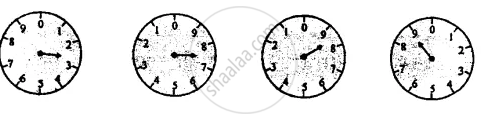Exercise 8 (A) (Short/Long answer questions) | Q 7 | Page 151

One day the meter reading is found to be 7643 units while the next day, it was 7657 units. What is the consumption of electricity in a day?

Exercise 8 (A) (Short/Long answer questions) | Q 8 | Page 151

A source of potential difference V volt sends current I ampere in a circuit for time t second. Write expressions for

1. electrical energy supplied by the source and
2. electrical power spent by the source.
Exercise 8 (A) (Short/Long answer questions) | Q 9 | Page 152

Name the unit in which you pay the cost of your electricity bill. How is it related to joule?

Exercise 8 (A) (Short/Long answer questions) | Q 10 | Page 152

If an appliance of power P watt is used for time t hour. How much electrical energy is consumed in kWh?

Exercise 8 (A) (Short/Long answer questions) | Q 11 | Page 152

What is an electric fuse? State its purpose in the household electrical circuit.

Exercise 8 (A) (Short/Long answer questions) | Q 12 | Page 152

State one property of the material of a fuse wire.

Exercise 8 (A) (Short/Long answer questions) | Q 13 | Page 152

Name the material of a fuse wire.

Exercise 8 (A) (Short/Long answer questions) | Q 14 | Page 152

Can we use copper wire as a fuse wire? Give reason.

Exercise 8 (A) (Short/Long answer questions) | Q 15 | Page 152

How does a fuse protect the electric wiring (or an appliance) from being damaged?

Exercise 8 (A) (Short/Long answer questions) | Q 16 | Page 152

Which fuse wire is thick: 5 A or 15 A?

Exercise 8 (A) (Short/Long answer questions) | Q 17 | Page 152

Write the full form of M.C.B?

Exercise 8 (A) (Short/Long answer questions) | Q 18 | Page 152

How is M.C.B. superior to the fuse wire?

Exercise 8 (A) (Short/Long answer questions) | Q 19 | Page 152

With which wire: live or neutral is the fuse wire connected?

Exercise 8 (A) (Short/Long answer questions) | Q 20 | Page 152

What do you mean by short-circuiting of a circuit?

Exercise 8 (A) (Short/Long answer questions) | Q 21 | Page 152

Figure shows two ways of connecting the three bulbs A, B and C to a battery. Name the two arrangements. Which of them do you prefer to use in a household circuit? Give a reason to support your answer.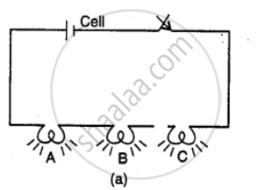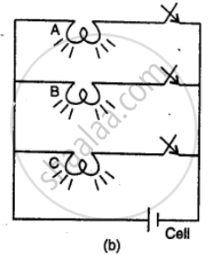Exercise 8 (A) (Short/Long answer questions) | Q 22 | Page 152

How are the electrical appliances connected in a house circuit: in series or in parallel? Give reason.

Exercise 8 (A) (Short/Long answer questions) | Q 23 | Page 152

In the household electric circuit, if one bulb is fused in a room, the other bulbs keep glowing. Explain the reason.

Exercise 8 (A) (Short/Long answer questions) | Q 24 | Page 152

State the voltage at which electricity is supplied to our houses.

Exercise 8 (A) (Short/Long answer questions) | Q 25

Draw a labelled diagram with the necessary switches to connect a bulb, a fan and a plug socket in a room with the mains. In what arrangement will you connect them to the mains?

Exercise 8 (A) (Short/Long answer questions) | Q 26 | Page 152

State the colour coding of the three wires in a cable used for

Exercise 8 (A) (Short/Long answer questions) | Q 27 | Page 152

Why is the metal covering of an electrical appliance earthed?

Exercise 8 (A) (Numericals) [Page 152]

### Selina solutions for Concise Physics Class 8 ICSE Chapter 8 Electricity Exercise 8 (A) (Numericals) [Page 152]

Exercise 8 (A) (Numericals) | Q 1.1 | Page 152

An electrical appliance is rated as 60 W – 150 V.
What do you understand by this statement?

Exercise 8 (A) (Numericals) | Q 1.2 | Page 152

An electrical appliance is rated as 60 W – 150 V.

How much current will flow through the appliance when in use?

Exercise 8 (A) (Numericals) | Q 2.1 | Page 152

An electric iron of power 1.5 kW is used for 30 minutes to press the clothes. Calculate the electrical energy consumed in kilowatt-hour

Exercise 8 (A) (Numericals) | Q 2.2 | Page 152

An electric iron of power 1.5 kW is used for 30 minutes to press the clothes. Calculate the electrical energy consumed in joule.

Exercise 8 (A) (Numericals) | Q 3 | Page 152

Assuming the electric consumption per day to be 12 kWh and the rate of electricity to be? 6.25 per unit, find how much money is to be paid in a month of 30 days?

Exercise 8 (A) (Numericals) | Q 4 | Page 152

In a premise 5 bulbs each of 100 W, 2 fans each of 60 W, 2 A.Cs each of 1.5 kW is used for 5 h per day. Find:
(a) Total power consumed per day,
(b) Total power consumed in 30 days,
(c) Total electrical energy consumed in 30 days.
(d) the cost of electricity at the rate of ₹ 6.25 per unit.

Exercise 8 (B) (Objectives question) [Pages 165 - 166]

### Selina solutions for Concise Physics Class 8 ICSE Chapter 8 Electricity Exercise 8 (B) (Objectives question) [Pages 165 - 166]

Exercise 8 (B) (Objectives question) | Q 1.01 | Page 165

The number of electrons and protons in an atom are the same.

• True

• False

Exercise 8 (B) (Objectives question) | Q 1.02 | Page 165

If the charge is not in motion, we call it static electricity.

• True

• False

Exercise 8 (B) (Objectives question) | Q 1.03 | Page 165

Human body is a conductor of electricity.

• True

• False

Exercise 8 (B) (Objectives question) | Q 1.04 | Page 165

When an ebonite rod is rubbed with fur, the electrons move from ebonite to fur.

• True

• False

Exercise 8 (B) (Objectives question) | Q 1.05 | Page 165

When glass rod is rubbed with dry silk cloth, the electrons move from glass to silk.

• True

• False

Exercise 8 (B) (Objectives question) | Q 1.06 | Page 165

The cap of gold leaf electroscope is made of copper.

• True

• False

Exercise 8 (B) (Objectives question) | Q 1.07 | Page 165

If a glass rod rubbed with silk is brought near the cap of a negatively charged electroscope, the divergence of leaves will decrease.

• True

• False

Exercise 8 (B) (Objectives question) | Q 1.08 | Page 165

In induction, a positively charged body can make an uncharged body positively charged.

• True

• False

Exercise 8 (B) (Objectives question) | Q 1.09 | Page 165

A lightning conductor saves the building from lighting.

• True

• False

Exercise 8 (B) (Objectives question) | Q 1.1 | Page 165

When a comb is rubbed with dry hair both comb and paper get similarly charged.

• True

• False

Exercise 8 (B) (Objectives question) | Q 1.11 | Page 165

A glass rod rubbed with silk repels an ebonite rod rubbed with fur.

• True

• False

Exercise 8 (B) (Objectives question) | Q 1.12 | Page 165

When an ebonite rod is rubbed with fur, the protons move from the ebonite rod to the fur.

• True

• False

Exercise 8 (B) (Objectives question) | Q 1.13 | Page 165

A conductor has a large number of free electrons.

• True

• False

Exercise 8 (B) (Objectives question) | Q 1.14 | Page 165

An ebonite rod can be charged by touching it with a charged copper rod.

• True

• False

Exercise 8 (B) (Objectives question) | Q 1.15 | Page 165

To find whether a body is charged or not, an uncharged electroscope is used.

• True

• False

Exercise 8 (B) (Objectives question) | Q 1.16 | Page 165

To find whether the charge on a body is positive or negative, an uncharged electroscope is used.

• True

• False

Exercise 8 (B) (Objectives question) | Q 1.17 | Page 165

If a negatively charged rod is brought near a negatively charged pith ball electroscope, the pith ball will be stuck with the rod.

• True

• False

Exercise 8 (B) (Objectives question) | Q 2.1 | Page 165

Like charges ............while unlike charges attract.

Exercise 8 (B) (Objectives question) | Q 2.2 | Page 165

Mercury is a ............ of electricity while pure water is ............ of electricity.

Exercise 8 (B) (Objectives question) | Q 2.3 | Page 165

An ebonite rod when rubbed with fur acquires the ............ charge.

Exercise 8 (B) (Objectives question) | Q 2.4 | Page 165

When an uncharged conductor is brought in contact with the disc of a gold leaf electroscope, its leaves ....................

Exercise 8 (B) (Objectives question) | Q 2.5 | Page 165

Charge is shared in charging a conductor by the method of ...............

Exercise 8 (B) (Objectives question) | Q 3 | Page 165

Match the following

 Column A Column B A. Two like charges 1 negative charge B. Two unlike charges 2 repel C. Silver is a 3 insulator D. Silk is an 4 attract E. Ebonite rod rubbed with fur acquires 5 conductor
Exercise 8 (B) (Objectives question) | Q 4.01 | Page 165

When a glass rod is rubbed with dry silk cloth, the charge acquired by the silk cloth is

• Positive

• negative

• both positive and negative

• none of the above

Exercise 8 (B) (Objectives question) | Q 4.02 | Page 166

When an ebonite rod is rubbed with fur, the rod acquires

• positive charge

• negative charge

• no charge

• none of the above

Exercise 8 (B) (Objectives question) | Q 4.03 | Page 166

When a negatively charged body is brought closer to another negatively charged body, then they will show

• attraction

• no effect

• repulsion

• none of the above

Exercise 8 (B) (Objectives question) | Q 4.04 | Page 166

Charging a conductor by bringing another charged conductor close to it without touching is called

• induction

• conduction

• convection

Exercise 8 (B) (Objectives question) | Q 4.05 | Page 166

The factor responsible for charging a conductor is

• transfer of protons

• transfer of neutrons

• transfer of electrons

• transfer of both protons and electrons

Exercise 8 (B) (Objectives question) | Q 4.06 | Page 166

Two objects when rubbed together get charged. The charges on them are

• equal and opposite

• equal and similar

• unequal and similar

• unequal and opposite

Exercise 8 (B) (Objectives question) | Q 4.07 | Page 166

When a glass rod is rubbed with silk, the glass rod and the silk get charged because

• electrons are transferred from the silk to the glass rod

• electrons are transferred from the glass rod to the silk

• protons are transferred from the silk to the glass rod

• protons are transferred from the glass rod to the silk

Exercise 8 (B) (Objectives question) | Q 4.08 | Page 166

The conductor of electricity is

• wood

• glass

• ebonite

• human body

Exercise 8 (B) (Objectives question) | Q 4.09 | Page 166

A gold-leaf electroscope is to be charged positively by conduction. For this

• a positively charged rod is held close to near the disc of electroscope

• a positively charged rod is placed in contact with the disc of electroscope

• a negatively charged rod is held near the disc of electroscope

• a negatively charged rod is touched with the disc of electroscope.

Exercise 8 (B) (Objectives question) | Q 4.1 | Page 166

A glass rod rubbed with silk is touched with the disc of a negatively charged gold leaf electroscope. The divergence of leaves will

• decrease

• increase

• remain unchanged

• first decrease and then increase.

Exercise 8 (B) (Objectives question) | Q 4.11 | Page 166

The rod in a gold leaf electroscope is made up of

• wood

• brass

• glass

• ebonite

Exercise 8 (B) (Objectives question) | Q 4.12 | Page 166

The lightning conductor is made up of:

• copper

• glass

• ebonite

• wood

Exercise 8 (B) (Short/Long answer questions) [Pages 166 - 168]

### Selina solutions for Concise Physics Class 8 ICSE Chapter 8 Electricity Exercise 8 (B) (Short/Long answer questions) [Pages 166 - 168]

Exercise 8 (B) (Short/Long answer questions) | Q 1 | Page 166

What do you understand by electricity at rest?

Exercise 8 (B) (Short/Long answer questions) | Q 2 | Page 166

Why does a plastic comb rubbed with dry hair attract bits of paper?

Exercise 8 (B) (Short/Long answer questions) | Q 3 | Page 166

Who discovered the way of producing electricity by friction?

Exercise 8 (B) (Short/Long answer questions) | Q 4 | Page 166

Name two substances which can be charged by friction.

Exercise 8 (B) (Short/Long answer questions) | Q 5 | Page 166

What are the two kinds of charges?

Exercise 8 (B) (Short/Long answer questions) | Q 6 | Page 166

A glass rod is rubbed with silk. State the kind of charge acquired by each.

Exercise 8 (B) (Short/Long answer questions) | Q 7 | Page 167

An ebonite rod is rubbed with fur. State the kind of-charge acquired by each.

Exercise 8 (B) (Short/Long answer questions) | Q 8 | Page 167

Describe an experiment to demonstrate that there are two r kinds of charges.

Exercise 8 (B) (Short/Long answer questions) | Q 9 | Page 167

How will you show that like charges repel and unlike charges attract each other?

Exercise 8 (B) (Short/Long answer questions) | Q 10 | Page 167

A glass rod rubbed with silk is suspended near an ebonite r rod rubbed with fur. What will be your observation? Give a reason to your answer.

Exercise 8 (B) (Short/Long answer questions) | Q 11 | Page 167

An ebonite rod rubbed with fur is suspended near another ebonite rod rubbed with fur. State your observation and give a reason to support your answer.

Exercise 8 (B) (Short/Long answer questions) | Q 12 | Page 167

What do you mean by conservation of charges?

Exercise 8 (B) (Short/Long answer questions) | Q 13 | Page 167

An ebonite rod is rubbed with fur. Compare the charges acquired by them.

Exercise 8 (B) (Short/Long answer questions) | Q 14 | Page 167

Name three constituents of an atom and state the kind of charge on each of them.

Exercise 8 (B) (Short/Long answer questions) | Q 15 | Page 167

What is the net charge on an atom?

Exercise 8 (B) (Short/Long answer questions) | Q 16 | Page 167

Briefly describe the structure of an atom.

Exercise 8 (B) (Short/Long answer questions) | Q 17 | Page 167

What are free electrons?

Exercise 8 (B) (Short/Long answer questions) | Q 18 | Page 167

What causes the charging of two objects when they are rubbed together?

Exercise 8 (B) (Short/Long answer questions) | Q 19 | Page 167

In each of the following cases, State which body loses electrons:
(a) A glass rod when rubbed with silk.
(b) An ebonite rod when rubbed with fur.

Exercise 8 (B) (Short/Long answer questions) | Q 20 | Page 167

A glass rod is rubbed with silk. Explain the charging of the glass rod and the silk on the basis of electron movement.

Exercise 8 (B) (Short/Long answer questions) | Q 21 | Page 167

An ebonite rod is rubbed with fur. Explain the charging of the ebonite rod and the fur on the basis of electron movement.

Exercise 8 (B) (Short/Long answer questions) | Q 22 | Page 167

Distinguish between conductors and insulators of electricity.

Exercise 8 (B) (Short/Long answer questions) | Q 23 | Page 167

Give one example each of a conductor and an insulator of electricity.

Exercise 8 (B) (Short/Long answer questions) | Q 24 | Page 167

State two ways of charging a conductor.

Exercise 8 (B) (Short/Long answer questions) | Q 25 | Page 167

Name the way of charging a conductor in which the charge is shared.

Exercise 8 (B) (Short/Long answer questions) | Q 26 | Page 167

Describe the method of charging a conductor by conduction.

Exercise 8 (B) (Short/Long answer questions) | Q 27 | Page 167

A metal rod A is to be charged positively by using another charged rod B. What should be the kind of charge on the rod B if charging is to be done by conduction?

Exercise 8 (B) (Short/Long answer questions) | Q 28 | Page 167

Explain the charging by conduction in terms of movement of electrons.

Exercise 8 (B) (Short/Long answer questions) | Q 29 | Page 167

Describe the method of charging a conductor by induction.

Exercise 8 (B) (Short/Long answer questions) | Q 30 | Page 167

Explain the charging by induction in terms of movement of electrons.

Exercise 8 (B) (Short/Long answer questions) | Q 31 | Page 167

Figure below shows a metal rod AB placed on an insulating stand.

In figure (a) a negatively charged ebonite rod C is touched with the metal rod AB, while in figure (b), the negatively charged ebonite rod C is held near the rod AB. State the kind of charges at the ends A and B of the rod, in each case.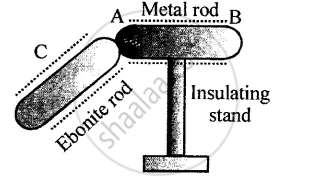Exercise 8 (B) (Short/Long answer questions) | Q 32 | Page 167

Can you charge an insulator by the method of conduction?

Exercise 8 (B) (Short/Long answer questions) | Q 33.1 | Page 167

What is an electroscope?

Exercise 8 (B) (Short/Long answer questions) | Q 33.2 | Page 167

Name the two types of electroscopes.

Exercise 8 (B) (Short/Long answer questions) | Q 34 | Page 167

Describe a pith ball electroscope. how can you use it to test whether a body is charged or uncharged?

Exercise 8 (B) (Short/Long answer questions) | Q 35 | Page 167

How will you use a pith ball electroscope to find out whether the charge on a charged body is positive or negative?

Exercise 8 (B) (Short/Long answer questions) | Q 36 | Page 167

Draw a labelled diagram of a gold leaf electroscope and describe its construction.

Exercise 8 (B) (Short/Long answer questions) | Q 37 | Page 168

A positively charged glass rod is touched with the disc of an uncharged gold leaf electroscope. What will be your observation?

Exercise 8 (B) (Short/Long answer questions) | Q 38 | Page 168

How will you use a gold leaf electroscope to find out whether a body is charged or uncharged?

Exercise 8 (B) (Short/Long answer questions) | Q 39 | Page 168

How will you use a gold leaf electroscope to find out whether the charge on a charged body is positive or negative?

Exercise 8 (B) (Short/Long answer questions) | Q 40 | Page 168

A negatively charged ebonite rod is touched with the disc of a negatively charged gold leaf electroscope. What will be your observation?

Exercise 8 (B) (Short/Long answer questions) | Q 41 | Page 168

When a charged rod is touched with the disc of a positively charged gold-leaf electroscope, it is observed that the divergence of leaves decreases. What is the kind of charge on the rod?

Exercise 8 (B) (Short/Long answer questions) | Q 42 | Page 168

Describe Franklin’s experiment. What did he conclude from his experiment?

Exercise 8 (B) (Short/Long answer questions) | Q 43 | Page 168

What causes lightning?

Exercise 8 (B) (Short/Long answer questions) | Q 44 | Page 168

What are the effects of lightning?

Exercise 8 (B) (Short/Long answer questions) | Q 45 | Page 168

What is a lightning conductor? How does it work?

Exercise 8 (B) (Short/Long answer questions) | Q 46 | Page 168

How is a tall building protected from damage due to lightning?

Exercise 8 (B) (Short/Long answer questions) | Q 47 | Page 168

State three safety measures that you will observe in thunder storm.

## Chapter 8: Electricity

Exercise 8 (A) (Objective questions)OthersExercise 8 (A) (Short/Long answer questions)Exercise 8 (A) (Numericals)Exercise 8 (B) (Objectives question)Exercise 8 (B) (Short/Long answer questions)## Selina solutions for Concise Physics Class 8 ICSE chapter 8 - Electricity

Selina solutions for Concise Physics Class 8 ICSE chapter 8 (Electricity) include all questions with solution and detail explanation. This will clear students doubts about any question and improve application skills while preparing for board exams. The detailed, step-by-step solutions will help you understand the concepts better and clear your confusions, if any. Shaalaa.com has the CISCE Concise Physics Class 8 ICSE solutions in a manner that help students grasp basic concepts better and faster.

Further, we at Shaalaa.com provide such solutions so that students can prepare for written exams. Selina textbook solutions can be a core help for self-study and acts as a perfect self-help guidance for students.

Concepts covered in Concise Physics Class 8 ICSE chapter 8 Electricity are Dangers of Electricity, Colour Coding of Live, Neutral, and Earth Wires, Earthing (Grounding), Precautions to Be Taken While Using Electricity, Electricity, Power Rating of Appliances, Electrical Energy and Power Consumed in a Circuit, Household Consumption of Electric Energy, Transmission of Power from the Power Generating Station to the Consumer, Miniature Circuit Breaker (MCB), Household Electrical Circuits, Electric Circuit in a Room, Electric Meter, Commercial Unit of Electrical Energy, Electric Fuse, Lightning and Lightning Safety, Static Electricity, Electric Charges, Conductors and Insulators, Methods of Charging a Conductor, Electroscope, Types of Charges and Their Interaction, Transfer of Charges, Lightning and Lightning Safety, Static Electricity, Electric Charges, Conductors and Insulators, Methods of Charging a Conductor, Electroscope, Types of Charges and Their Interaction, Transfer of Charges.

Using Selina Class 8 solutions Electricity exercise by students are an easy way to prepare for the exams, as they involve solutions arranged chapter-wise also page wise. The questions involved in Selina Solutions are important questions that can be asked in the final exam. Maximum students of CISCE Class 8 prefer Selina Textbook Solutions to score more in exam.

Get the free view of chapter 8 Electricity Class 8 extra questions for Concise Physics Class 8 ICSE and can use Shaalaa.com to keep it handy for your exam preparation